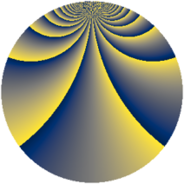# Properties

 Label 1840.2.ctLevel $1840$ Weight $2$ Character orbit 1840.ct Rep. character $\chi_{1840}(17,\cdot)$ Character field $\Q(\zeta_{44})$ Dimension $1400$ Sturm bound $576$

# Related objects

## Defining parameters

 Level: $$N$$ $$=$$ $$1840 = 2^{4} \cdot 5 \cdot 23$$ Weight: $$k$$ $$=$$ $$2$$ Character orbit: $$[\chi]$$ $$=$$ 1840.ct (of order $$44$$ and degree $$20$$) Character conductor: $$\operatorname{cond}(\chi)$$ $$=$$ $$115$$ Character field: $$\Q(\zeta_{44})$$ Sturm bound: $$576$$

## Dimensions

The following table gives the dimensions of various subspaces of $$M_{2}(1840, [\chi])$$.

Total New Old
Modular forms 6000 1480 4520
Cusp forms 5520 1400 4120
Eisenstein series 480 80 400

## Trace form

 $$1400q + 18q^{3} - 22q^{5} + 22q^{7} + O(q^{10})$$ $$1400q + 18q^{3} - 22q^{5} + 22q^{7} + 44q^{11} - 18q^{13} + 22q^{15} - 22q^{17} - 44q^{21} + 32q^{23} - 18q^{25} + 54q^{27} + 20q^{31} - 22q^{33} + 18q^{35} - 22q^{37} - 52q^{41} + 22q^{43} + 64q^{47} + 44q^{51} - 22q^{53} + 86q^{55} - 22q^{57} - 44q^{61} + 22q^{63} - 22q^{65} + 22q^{67} + 36q^{71} - 18q^{73} + 126q^{75} - 46q^{77} + 56q^{81} + 22q^{83} - 18q^{85} + 78q^{87} + 4q^{93} + 214q^{95} - 22q^{97} + O(q^{100})$$

## Decomposition of $$S_{2}^{\mathrm{new}}(1840, [\chi])$$ into newform subspaces

The newforms in this space have not yet been added to the LMFDB.

## Decomposition of $$S_{2}^{\mathrm{old}}(1840, [\chi])$$ into lower level spaces

$$S_{2}^{\mathrm{old}}(1840, [\chi]) \cong$$ $$S_{2}^{\mathrm{new}}(115, [\chi])$$$$^{\oplus 5}$$$$\oplus$$$$S_{2}^{\mathrm{new}}(230, [\chi])$$$$^{\oplus 4}$$$$\oplus$$$$S_{2}^{\mathrm{new}}(460, [\chi])$$$$^{\oplus 3}$$$$\oplus$$$$S_{2}^{\mathrm{new}}(920, [\chi])$$$$^{\oplus 2}$$